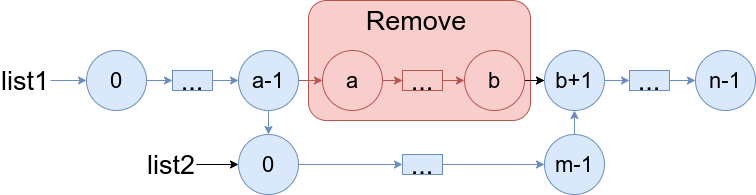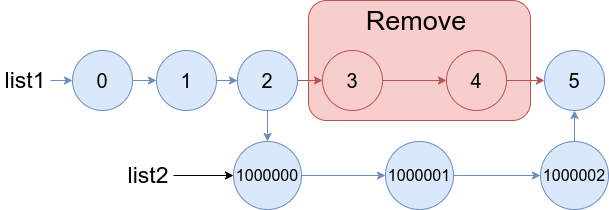# 1669. Merge In Between Linked Lists | LeetCode Solution

## 1669. Merge In Between Linked Lists | LeetCode Solution

You are given two linked lists: `list1` and `list2` of sizes `n` and `m` respectively.

Remove `list1`'s nodes from the `ath` node to the `bth` node, and put `list2` in their place.

The blue edges and nodes in the following figure indicate the result:Build the result list and return its head.

Example 1:```Input: list1 = [0,1,2,3,4,5], a = 3, b = 4, list2 = [1000000,1000001,1000002]
Output: [0,1,2,1000000,1000001,1000002,5]
Explanation: We remove the nodes 3 and 4 and put the entire list2 in their place. The blue edges and nodes in the above figure indicate the result.
```

Example 2:```Input: list1 = [0,1,2,3,4,5,6], a = 2, b = 5, list2 = [1000000,1000001,1000002,1000003,1000004]
Output: [0,1,1000000,1000001,1000002,1000003,1000004,6]
Explanation: The blue edges and nodes in the above figure indicate the result.
```

Constraints:

• `3 <= list1.length <= 104`
• `1 <= a <= b < list1.length - 1`
• `1 <= list2.length <= 104`

/**
* struct ListNode {
*     int val;
*     ListNode *next;
*     ListNode() : val(0), next(nullptr) {}
*     ListNode(int x) : val(x), next(nullptr) {}
*     ListNode(int x, ListNode *next) : val(x), next(next) {}
* };
*/
class Solution {
public:
ListNode* mergeInBetween(ListNode* list1, int a, int b, ListNode* list2) {
int m=a,n=b;
ListNode* ans=list1;
while(a>1){
list1=list1->next;
a--;
}
while(b>0){